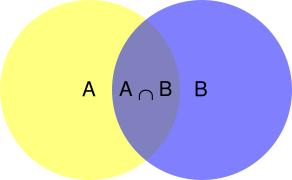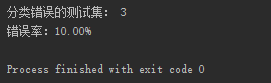# 个人博客转至：tybai.com# 【机器学习】算法原理详细推导与实现(三):朴素贝叶斯

## 贝叶斯定理

### 定理推导$p(A|B)=\frac{p(A\bigcap B)}{p(B)}$

$p(B|A)=\frac{p(B\bigcap A)}{p(A)}$

$p(A|B)p(B)=p(A\bigcap B)=p(B|A)p(A)$

$P(A|B) = \frac{P(B|A)P(A)}{P(B)}$

1. 贝叶斯定理是将先验概率做一次更新，得到后验概率
2. 朴素贝叶斯是输入先验概率，找到后验概率，最后找到最大后验概率，作为最终的分类结果，以及分类概率

### 解决方案

$P(x1|y) = \frac{P(y|x1)P(x1)}{P(y)}$

$\begin{split} P(x1|y)&=\frac{P(y|x1)P(x1)}{P(y)} \\ &=\frac{P(y|x1)P(x1)}{P(y|x1)P(x1)+ P(y|x2)P(x2)} \\ &= \frac{0.75\times0.5}{0.75\times0.5+0.5\times0.5} \\ &=0.6 \end{split}$

## 朴素贝叶斯

word_1
word_2
word_3
...
word_n

$x= \begin{bmatrix} 1 \\ 1 \\ 0 \\ ... \\ 1 \end{bmatrix}$

## 算法推导

$\begin{split} p(x_1,x_2,...,x_{50000}|y)&=p(x_1|y)p(x_1|y,x_1)p(x_2|y,x_1,x_2)...p(x_50000|y,x_1,x_2,...,x_{49999}) \\ &=p(x_1|y)p(x_2|y)...p(x_{50000}|y) \\ &=\prod_{i=1}^n p(x_i|y) \end{split}$

$\phi_{i|y=1}=p(x_i=1|y=1) \phi_{i|y=0}=p(x_i=1|y=0) \phi_y=p(y=1)$

$L(\phi_y,\phi_{i|y=0},\phi_{i|y=1})=\prod_{i=1}^m p(x^{(i)}|y^{(i)})$

$\phi_{j|y=1}=\frac{\sum_{i=1}^ml\{x_j^{(i)}\bigcap y^{(i)}=1\}}{\sum_{i=1}^ml\{y^{(i)}=1\}}$

$\phi_{j|y=0}=\frac{\sum_{i=1}^ml\{x_j^{(i)}=1\bigcap y^{(i)}=0\}}{\sum_{i=1}^ml\{y^{(i)}=0\}}$

$\phi_{j|y=1}=\frac{\sum_{i=1}^ml\{x_j^{(i)}=1\bigcap y^{(i)}=1\}}{\sum_{i=1}^ml\{y^{(i)}=1\}}$

$(x^{(1)},y^{(1)}),(x^{(2)},y^{(2)}),...,(x^{(m)},y^{(m)})$

$\begin{split} p(y=1|x)&=\frac{p(x|y=1)p(y=1)}{p(x)} \\ &=\frac{(\prod_{i=1}^n p(x_i|y=1))p(y=1)}{(\prod_{i=1}^n p(x_i|y=1))p(y=1)+(\prod_{i=0}^n p(x_i|y=0))p(y=0)} \end{split}$

$\begin{split} p(y=1|x)&=\frac{p(x|y=1)p(y=1)}{p(x)} \\ &=\frac{(\prod_{i=1}^{50000} p(x_3|y=1))p(y=1)}{(\prod_{i=1}^{50000} p(x_3|y=1))p(y=1)+(\prod_{i=0}^n p(x_i|y=0))p(y=0)} \\ &=\frac{0}{0+0} \end{split}$

### 拉普拉斯平滑(Laplace smoothing)

$\begin{split} p(y=0)&=\frac{没投中的次数}{投篮球的总次数} \\ &=\frac{没投中的次数}{投中的次数+没投中的次数} \\ &=\frac{0}{5+0} \end{split}$

$\begin{split} p(y=0)&=\frac{0+1}{(5+1)+(0+1)} \\ &=\frac{1}{7} \end{split}$

$\phi_{j|y=1}=\frac{\sum_{i=1}^ml\{x_j^{(i)}\bigcap y^{(i)}=1\}+1}{\sum_{i=1}^ml\{y^{(i)=1}\}+2}$

$\phi_{j|y=0}=\frac{\sum_{i=1}^ml\{x_j^{(i)}=1\bigcap y^{(i)}=0\}+1}{\sum_{i=1}^ml\{y^{(i)}=0\}+2}$

### 总结

$p(x|y)=(\prod_{i=1}^{n}p(x_i|y_i)$

$argmax_yp(y|x)=argmax_y \frac{p(x|y)p(y)}{p(x)}argmax_y p(x|y)p(y)$

## 实例

# 打开数据集，获取邮件内容，
# spam为垃圾邮件，ham为正常邮件
# 选取一部分邮件作为测试集
testIndex = random.sample(range(1, 25), 5)

dict_word_temp = []

testList = []
trainList = []
testLabel = []
trainLabel = []

for i in range(1, 26):
wordListSpam = textParse(open('./email/spam/%d.txt' % i, 'r').read())
wordListHam = textParse(open('./email/ham/%d.txt' % i, 'r').read())
dict_word_temp = dict_word_temp + wordListSpam + wordListHam

if i in testIndex:
testList.append(wordListSpam)
# 用1表示垃圾邮件
testLabel.append(1)
testList.append(wordListHam)
# 用0表示正常邮件
testLabel.append(0)
else:
trainList.append(wordListSpam)
# 用1表示垃圾邮件
trainLabel.append(1)
trainList.append(wordListHam)
# 用0表示正常邮件
trainLabel.append(0)

# 去重得到词字典
dict_word = list(set(dict_word_temp))
trainData = tranWordVec(dict_word, trainList)
testData = tranWordVec(dict_word, testList)

return trainData, trainLabel, testData, testLabel

# 训练函数
def train(trainData, trainLabel):
trainMatrix = np.array(trainData)

# 计算训练的文档数目
trainNum = len(trainMatrix)
# 计算每篇文档的词条数
wordNum = len(trainMatrix)
# 文档属于垃圾邮件类的概率
ori_auc = sum(trainLabel) / float(trainNum)

# 拉普拉斯平滑
# 分子+1
HamNum = np.ones(wordNum)
SpamNum = np.ones(wordNum)
# 分母+2
HamDenom = 2.0
SpamDenom = 2.0

for i in range(trainNum):
# 统计属于垃圾邮件的条件概率所需的数据，即P(x0|y=1),P(x1|y=1),P(x2|y=1)···
if trainLabel[i] == 1:
SpamNum += trainMatrix[i]
SpamDenom += sum(trainMatrix[i])
else:
# 统计属于正常邮件的条件概率所需的数据，即P(x0|y=0),P(x1|y=0),P(x2|y=0)···
HamNum += trainMatrix[i]
HamDenom += sum(trainMatrix[i])
# 取对数，防止下溢出
SpamVec = np.log(SpamNum / SpamDenom)
HamVec = np.log(HamNum / HamDenom)
# 返回属于正常邮件类的条件概率数组，属于垃圾邮件类的条件概率数组，文档属于垃圾邮件类的概率
return HamVec, SpamVec, ori_auc

# 预测函数
def predict(testDataVec, HamVec, SpamVec, ori_auc):
predictToSpam = sum(testDataVec * SpamVec) + np.log(ori_auc)
predictToHam = sum(testDataVec * HamVec) + np.log(1.0 - ori_auc)
if predictToSpam > predictToHam:
return 1
else:
return 0posted on 2019-07-05 10:09  TTyb  阅读(...)  评论(...编辑  收藏

### 导航